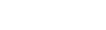﻿ TOraInterval.Compare MethodODAC

# TOraInterval.Compare MethodCompares the current TOraInterval value with the Dest value.

### Syntax

function Compare(Dest: TOraInterval): integer;

Parameters
Dest
Holds the Dest value.
Return Value
the negative value if current interval is shorter than Dest, zero if intervals are equal, and positive value if the current interval is longer than Dest.

### Remarks

Call the Compare method to compare the current TOraInterval value with Dest value.

Returns negative value if current interval is shorter than Dest, zero if intervals are equal and positive value if current interval is longer than Dest.